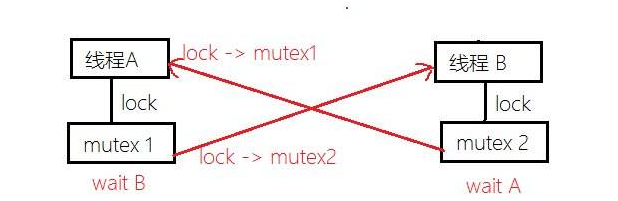浅谈互斥锁与进程间的通信 (举例说明)ShenDu_Linux一、互斥锁

﻿

1.上厕所的小例子：你上厕所的时候肯定得锁门吧，有人来了看见门锁着，就会在外面等着，等你吧门开开出来的时候，下一个人才去上厕所。

﻿

from multiprocessing import Process,Lock
import os
import time
def work(mutex):
mutex.acquire()
time.sleep(3)
mutex.release()
if __name__ == '__main__':
mutex = Lock()
p1 = Process(target=work,args=(mutex,))
p2 = Process(target=work,args=(mutex,))
p3 = Process(target=work,args=(mutex,))
p1.start()
p2.start()
p3.start()
p1.join()
p2.join()
p3.join()
print('主')

二、模拟抢票（也是利用了互斥锁的原理 ：LOCK互斥锁）

﻿

import json
import time
from multiprocessing import Process,Lock
def search(name):
time.sleep(1)
print("<%s> 查看到的剩余票数 %s" % (name, dic["count"]))
def get(name):
time.sleep(0.1)
if dic["count"] > 0:
dic["count"] -= 1
time.sleep(0.1)
json.dump(dic, open("db.txt", "w", encoding="utf-8"))
print("<%s> 购票成功" % name)
else:
print("<%s> 购票失败，已经无剩余票" % name)
search(name)
mutex.acquire()
get(name)
mutex.release()
if __name__ == "__main__":
mutex = Lock()
for i in range(100):
p = Process(target=task, args=("路人%s" % i, mutex))
p.start()

﻿

三、Process对象的其他属性

﻿

1. p.daemon ：守护进程（必须在开启之前设置守护进程）：如果父进程死，子进程p也死了

2. p.join：父进程等p执行完了才运行主进程，是父进程阻塞在原地，而p仍然在后台运行。

3. terminate：强制关闭。（确保p里面没有其他子进程的时候关闭，如果里面有子进程，你去用这个方法强制关闭了就会产生僵尸进程（打个比方：如果你老子挂了，你还没挂，那么就没人给你收尸了，啊哈哈））

4. is_alive：关闭进程的时候,不会立即关闭,所以is_alive立刻查看的结果可能还是存活

5. p.join()：父进程在等p的结束，是父进程阻塞在原地，而p仍然在后台运行

6. p.name：查看名字

7. p.pid ：查看id

﻿

四、进程间的三种通信（IPC）方式

﻿

﻿

﻿

1.队列：队列类似于一条管道，元素先进先出

2.队列分类

﻿

queue.Queue() #先进先出

queue.LifoQueue() #后进先出

queue.PriorityQueue() #优先级队列

queue.deque() #双线队列

﻿

﻿

Queue([maxsize]):创建共享的进程队列，Queue是多进程安全的队列，

﻿

﻿

1 maxsize是队列中允许最大项数，省略则无大小限制。

﻿

﻿

q.put方法用以插入数据到队列中，put方法还有两个可选参数：
blocked和timeout。如果blocked为True（默认值），并且timeout为正值，该方法会阻塞timeout指定的时间，

q.get方法可以从队列读取并且删除一个元素。

q.get_nowait():同q.get(False)
q.put_nowait():同q.put(False)
q.empty():调用此方法时q为空则返回True，该结果不可靠，比如在返回True的过程中，

q.full()：调用此方法时q已满则返回True，该结果不可靠，比如在返回True的过程中，

q.qsize():返回队列中目前项目的正确数量，结果也不可靠，理由同q.empty()和q.full()一样

﻿

﻿

# 1.可以往队列里放任意类型的
# 2.先进先出
from multiprocessing import Process,Queue
q= Queue(3)
q.put('first') #默认block=True
q.put('second')
q.put('third')
print(q.get())
print(q.get())
print(q.get())

﻿

﻿

﻿

﻿

﻿

﻿

﻿

﻿

﻿

1、q.put(None)：生产者给放一个None进去

﻿

from multiprocessing import Process,Queue
import os
import time
import random
#首先得有生产者和消费者
# 生产者制造包子
'''这种用 q.put(None)放进去一个None的方法虽然解决了问题

def producter(q):
for i in range(10):
time.sleep(2) #生产包子得有个过程，就先让睡一会
res = '包子%s'%i #生产了这么多的包子
q.put(res) #把生产出来的包子放进框里面去
print('\033[44m%s制造了%s\033[0m'%(os.getpid(),res))
q.put(None) #只有生产者才知道什么时候就生产完了（放一个None进去说明此时已经生产完了）
# 消费者吃包子
def consumer(q):
while True:#假如消费者不断的吃
res = q.get()
if res is None:break #如果吃的时候框里面已经空了，就直接break了
time.sleep(random.randint(1,3))
print('\033[41m%s吃了%s\033[0m' % (os.getpid(),res))
if __name__ == '__main__':
q = Queue()
p1 = Process(target=producter,args=(q,))
p2 = Process(target=consumer,args=(q,))
p1.start()
p2.start()
p1.join()
p2.join() #等待执行完上面的进程，在去执行主
print('主')

﻿

2、利用JoinableQueue

﻿

from multiprocessing import Process,JoinableQueue
import os
import time
import random
#首先得有生产者和消费者
# 消费者吃包子
def consumer(q):
while True:
res = q.get()
time.sleep(random.randint(1,3))
print('\033[41m%s吃了%s\033[0m' % (os.getpid(),res))
def product_baozi(q):
for i in range(5):
time.sleep(2)
res = '包子%s' % i
q.put(res)
print('\033[44m%s制造了%s\033[0m' % (os.getpid(), res))
q.join() #不用put(None) 了，在等q被取完。（如果数据没有被取完，生产者就不会结束掉）
def product_gutou(q):
for i in range(5):
time.sleep(2)
res = '骨头%s' % i
q.put(res)
print('\033[44m%s制造了%s\033[0m' % (os.getpid(), res))
q.join()
def product_doujiang(q):
for i in range(5):
time.sleep(2)
res = '豆浆%s' % i
q.put(res)
print('\033[44m%s制造了%s\033[0m' % (os.getpid(), res))
q.join()
if __name__ == '__main__':
q = JoinableQueue()
# 生产者们：厨师们
p1 = Process(target=product_baozi,args=(q,))
p2 = Process(target=product_doujiang,args=(q,))
p3 = Process(target=product_gutou,args=(q,))
#消费者们：吃货们
p4 = Process(target=consumer,args=(q,))
p5 = Process(target=consumer,args=(q,))
p4.daemon = True
p5.daemon = True
# p1.start()
# p2.start()
# p3.start()
# p4.start()
# p5.start()
li = [p1,p2,p3,p4,p5]
for i in li:
i.start()
p1.join()
p2.join()
p3.join()
print('主')

﻿

﻿

﻿

﻿

﻿

﻿

Epoll的具体实现与epoll线程安全，互斥锁，自旋锁，CAS，原子操作

﻿评论